Using a simple voltage divider circuit, you can use the analog input 5 (DC 0..16V) of the D105 to connect a wide variety of sensors:

• DC voltage measurement above 16V;
• resistive sensors (temperature, level, photoresistors, etc);

#### The general voltage divider circuit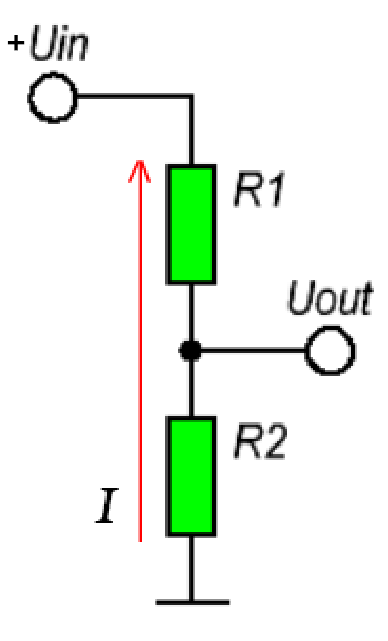The voltage divider circuit includes an input voltage source Uin and two resistors R1 and R2. To find out what voltage will be at the output of the divider Uout, we use Ohm's law.Assume the values ​​are:Uin = 5 V; (for example, + 5V we take from terminal 6)R1 = 110 ohms;R2 = 390 ohms.According to Ohm's law, we calculate the total current flowing through the entire circuit, i.e. through two series-connected resistors.Then, by the same Ohm's law, we determine the voltage Uout on the resistor R2.
```Ohm's law: I = U / R
For series connected resistors: R = R1 + R2
Общий ток:
I = Uin / R = 5V / (110 ohm + 390 ohm) = 0.01 A

Voltage Uout on resistor R2, according to Ohm's law: U = I * R
Uout = I * R2 = 0.01 A * 390 ohm = 3.9 V
```

Everything is simple, and there is also a ready-made formula for calculating the voltage Uout at the output of the divider: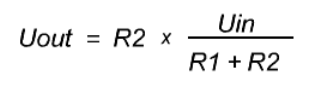#### Specificity when connecting to D105

ADC analog input (5) is designed to measure voltage within 0 .. 16V for direct current (DC).
For the ability to measure voltages above 16V, it is possible to use a simple voltage divider.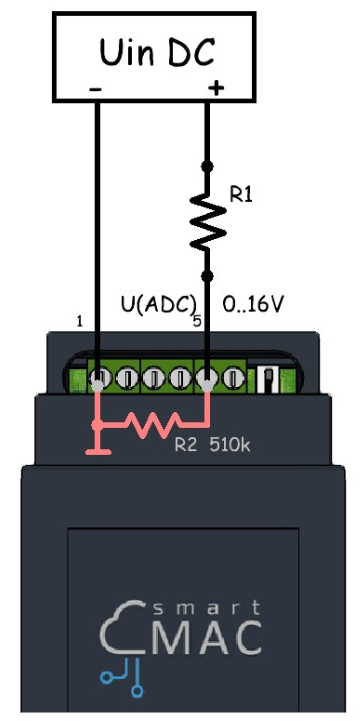When calculating the voltage divider, it is enough to calculate the resistance R1, assuming that inside the device there is a constant resistance R2, which is 510 k.The formula for calculating the voltage V (ADC) at the analog input (5) ADC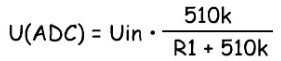The formula for selecting resistance R1, in accordance with the input voltage Vin and the desired V (ADC)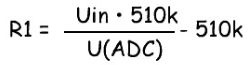Voltage divider ratio: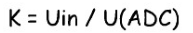The voltage divider ratio is used in the widget settings, in the "Arithmetic operation" section multiplied by the divider coefficient.

#### Example of connecting a Level Sensor

When connecting a level sensor with several gradations of measurements, using a simple voltage divider, you can obtain different voltages for different measurement levels at the analog input (5).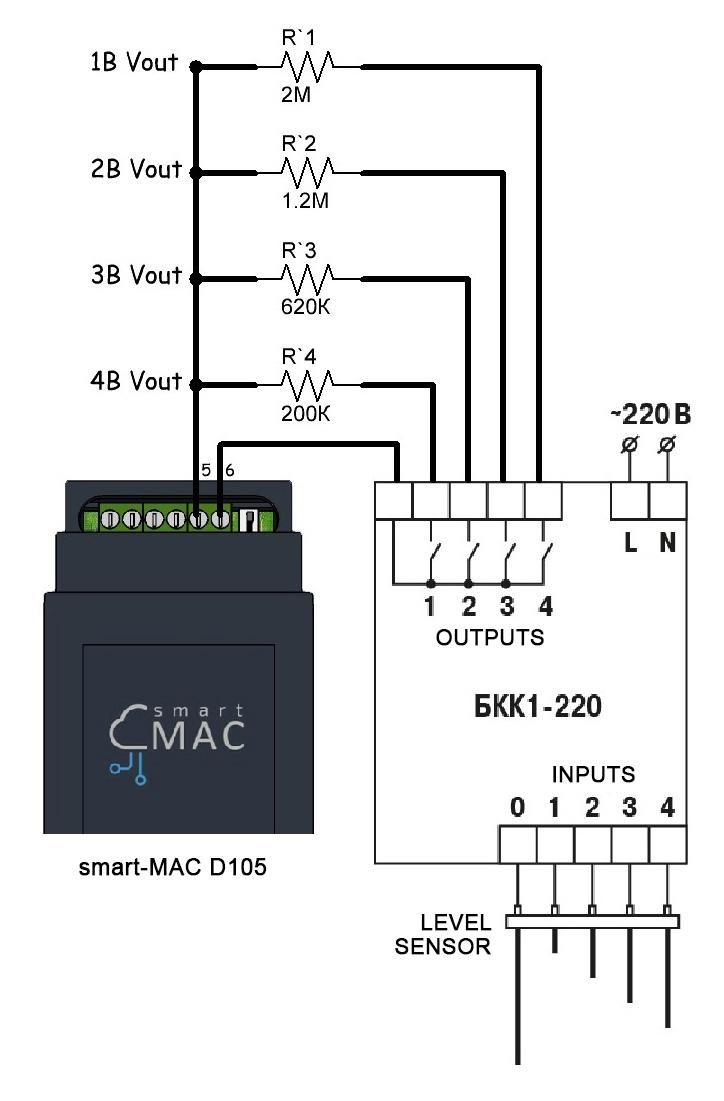For example, we have a Level Sensor for a tank with 4 gradations of depth 1-2-3-4 meters. At a certain level at the INPUT, the corresponding contact groups at the OUTPUT will be switched on.

The resistance of the voltage divider was selected in such a way that at a level of 1-2-3-4 meters, the corresponding voltage of 1-2-3-4V will be at the input (5).

- With a depth of 1 meter (input 4), the output 4 will switch on and as a result we have a simple voltage divider:

```R1 = "R1-1"
Uout = 510 * (5V / (2M + 510k)) = 1V```

- With a depth of 2 meters, outputs 4 and 3 will switch on. The resistance R1 will be calculated as the parallel connection of the resistors "R1-1" and "R1-2" and as a result we have a voltage divider:

```For parallel-connected resistors:
R1 = 1 / (1/R1-1 + 1/R1-2) = 750k
Uout = 510 * (5V / (750k + 510k)) = 2V```

- With a depth of 3 meters, outputs 4, 3 and 2 will switch on. Resistance R1 will be calculated as the parallel-connection of resistors "R1-1", "R1-2" and "R1-3".

```R1 = 1 / (1/2000 + 1/1200 + 1/620) = 340k
Uout = 510 * (5V / (340k + 510k)) = 3V```

And so on for any number of gradations of measurements.

#### Connecting resistive sensors with low resistance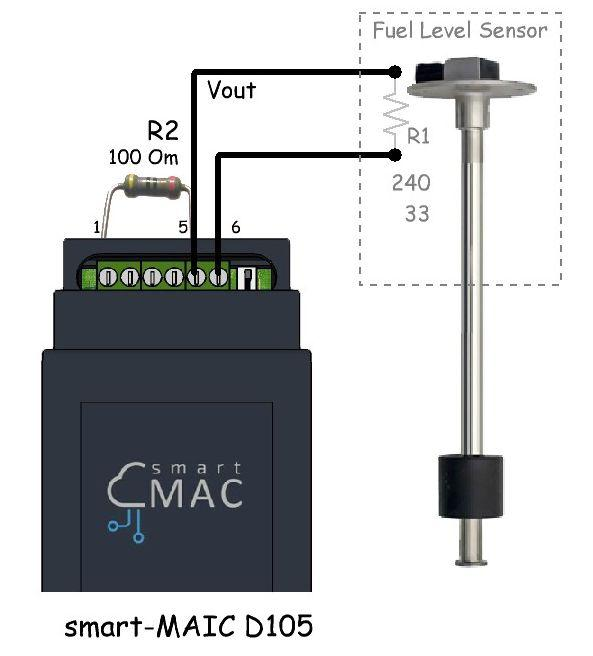When using resistive sensors with a variable internal resistance of up to 1 kOhm, when calculating the voltage divider, the internal resistance of 510 kOhm in the device can be neglected.

For example, we have a Fuel Level Sensor in the tank, which works according to the American standard with a change in internal resistance within 240 .. 33 Ohms.
Let calculate the lower and upper values ​​for the voltage divider according to the standard scheme.
Taking the following input:

Uin = 5 V (take from terminal 6)

R1 = от 240 ohm до 33 ohm

R2 = 100 ohm

```The general voltage divider formula:
Uout = R2*(Uin/(R1+R2))

- when R1=240 ohm
Uout = 100*(5/(240+100)) = 1.5 V

- when R1=33 ohm
Uout = 100*(5/(33+100)) = 3.8 V```

As a result, we have a linear dependence of the voltage and the fuel level: 1.5V at 0% level and 3.8 V at a 100% level.

To display the fuel level on the widget, in the settings in the "Arithmetic operation" section select the function f (x) and write the formula for converting the voltage to the fuel level.

In percent [%], for 1V is: 100% / (3.8 - 1.5) = 43.48%

`d.ADC > 1.5 ? (d.ADC-1.5)*43.48 : 0`

In liters, knowing the volume of the tank, for example, 200 liters [lites].

For 1V is: 200 liters / (3.8 - 1.5) = 86.96 lites

`d.ADC > 1.5 ? (d.ADC-1.5)*86.96 : 0`

Memo for calculating the voltage divider for the universal meter smart-MAC D105

d105 Voltage divider_EN.JPG